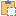#### BoundingBoxUV Class

A two-dimensional rectangle, parallel to the coordinate axes.

Namespace: Autodesk.Revit.DB
Assembly: RevitAPI (in RevitAPI.dll) Version: 17.0.0.0 (17.0.1090.0)

# Syntax

C#
``public class BoundingBoxUV : IDisposable``
Visual Basic
``````Public Class BoundingBoxUV _
Implements IDisposable``````
Visual C++
``public ref class BoundingBoxUV : IDisposable``

# ExamplesCopy C#
``````Document doc = application.ActiveUIDocument.Document;
FilteredElementCollector collector = new FilteredElementCollector(doc);
ICollection<Element> collection = collector.OfClass(typeof(Autodesk.Revit.DB.View)).ToElements();
foreach (Element element in collection)

{
Autodesk.Revit.DB.View view = element as Autodesk.Revit.DB.View;
if (null != view)
{
string messageInfo = "BoundingBoxUV : ";
messageInfo += "\nView name : " + view.Name;
BoundingBoxUV boundingBox = view.Outline;
// Get max bounding box
UV max = boundingBox.Max;
messageInfo += "\nMax boundingbox : (" + max.U + "," + max.V + ")";
// Get min bounding box
UV min = boundingBox.Min;
messageInfo += "\nMin boundingbox : (" + min.U + "," + min.V + ")";
// Get bounds,
// Use 0 for Min and 1 for Max
UV bound = boundingBox.get_Bounds(0);
messageInfo += "\nBoundingbox bounds : (" + bound.U + "," + bound.V + ")";
}
}``````Copy VB.NET
``````Dim doc As Document = application.ActiveUIDocument.Document
Dim collector As New FilteredElementCollector(doc)
Dim collection As ICollection(Of Element) = collector.OfClass(GetType(Autodesk.Revit.DB.View)).ToElements()
For Each element As Element In collection

Dim view As Autodesk.Revit.DB.View = TryCast(element, Autodesk.Revit.DB.View)
If view IsNot Nothing Then
Dim messageInfo As String = "BoundingBoxUV : "
messageInfo += vbLf & "View name : " + view.Name
Dim boundingBox As BoundingBoxUV = view.Outline
' Get max bounding box
Dim max As UV = boundingBox.Max
messageInfo += vbLf & "Max boundingbox : (" + max.U + "," + max.V + ")"
' Get min bounding box
Dim min As UV = boundingBox.Min
messageInfo += vbLf & "Min boundingbox : (" + min.U + "," + min.V + ")"
' Get bounds,
' Use 0 for Min and 1 for Max
Dim bound As UV = boundingBox.Bounds(0)
messageInfo += vbLf & "Boundingbox bounds : (" + bound.U + "," + bound.V + ")"